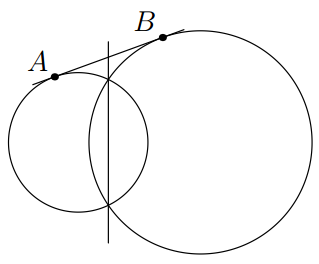PowerOfPointTheorem

Problem - 3142

Let $O_1$ and $O_2$ be two intersecting circles. Let a common tangent to these two circles touch $O_1$ at $A$ and $O_2$ at $B$. Show that the common chord of these two circles, when extended, bisects segment $AB$.